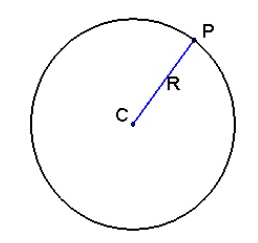Q

# Tell me? A wire of length 2 units is cut into two parts which are bent respectively to form a square of side=x units and a circle of radius=r units. If the sum of the areas of the square and the circle so formed is minimum, then :

A wire of length 2 units is cut into two parts which are bent respectively to form a square of side=x units and a circle of radius=r units.  If the sum of the areas of the square and the circle so formed is minimum, then :

• Option 1)

2x = (π+4) r

• Option 2)

(4−π) x = πr

• Option 3)

x = 2r

• Option 4)

2x = r

523 Views
N

As we learnt in

Circle -

A circle is the locus of a moving point such that its distance from a fixed point is constant.

- whereinLet the length of two parts be 'a' and '2-a'

and

where x is side of square

sum of Areas

of

Hence

Option 1)

2x = (π+4) r

Incorrect

Option 2)

(4−π) x = πr

Incorrect

Option 3)

x = 2r

Correct

Option 4)

2x = r

Incorrect

Exams
Articles
Questions